Register for Maths, Science, English, Reasoning Olympiad Exams Register here | Book Free Demo for Live Olympiad Classes here| Check Olympiad Exam Dates here | Buy Practice Papers for IMO, IOM, HEO, IOEL etc here | Login here to participate in all India free mock test on every Saturday

# Force and Pressure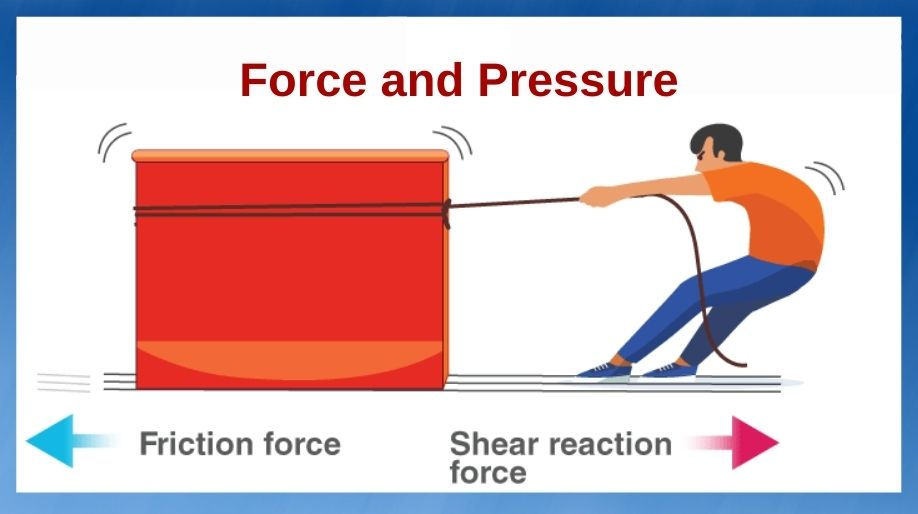## Force

• Force is said to be a pull or push.
• Force can be applied by living and non-living things.
• Source of force and object have to interact to apply a force to an object.
• Between the two objects, one applies the force and the other objects receive the force.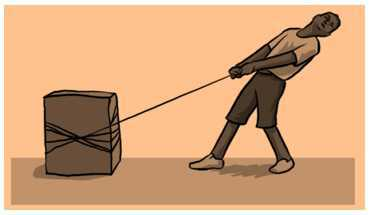## Direction and magnitude of force

• Magnitude of force is the amount of force and measurement of strength.
• The force can be applied to two objects in the same direction and opposite direction.

## Effect of forces

1. State of motion can be changes with the force
• Rest State - Rest state means the object is not moving.
• Motion state – When an object is moving, then it is said to be in motion state. A force can bring an object in motion state from rest state.

Some examples are

• If a tyre by a boy is to be moved then he will have to push it repeatedly.
• In the game of football, the goalkeeper stops the ball reaching the court by applying the force.
1. The shape of an object can be changed with the force.
• Shape of a rubber band can be changed by stretching it in the opposite direction.

## Types of Force

1. Contact Force
• It is necessary for the objects to interact.
• This force keeps working on the point of contact.
• Friction force and muscular force are the two types of contact force.
• Examples are hitting the ball, pushing a car, Kicking a ball, etc.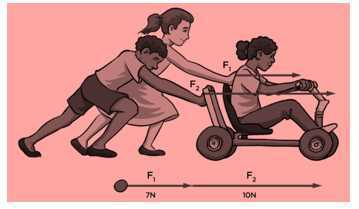1. Non-Contacting force
• There is no interaction between the two objects in this force.
• Electrostatic force, Magnetic force and Gravitational force are the examples of non-contact force.
• Example is pulling of an iron nail by a magnet from a distance.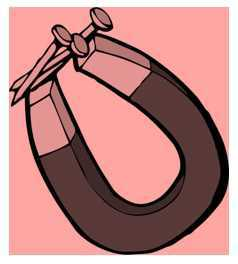## Pressure

• Pressure is the force put on per unit area of any surface.
• Pressure = Force/Area
• Pressure and Area are indirectly proportional.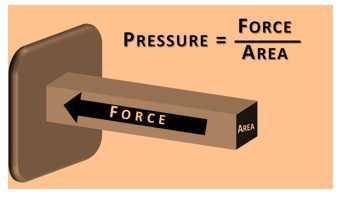## Pressure exerted by Liquid and Gas

• The inner wall of a container which keeps Liquid and gas gets affected by their pressure.
• The balloon gets inflated when the air gets filled due to the pressure.
• Due to the pore in the bottle, the water starts leaking. This happens because Walls of the bottle gets pressure from the water.

### Atmospheric Pressure

• Different types of gases together make our atmosphere. Hence when the pressure is exerted by the gases it gets exerted in the air and then it is called as Atmospheric pressure.
• Due to the effect of atmospheric pressure on a plane the pressed rubber sucker does not come off.

## Recap

• Force is said to be a pull or push.
• Force can be applied by living and non-living things.
• Magnitude of force is the amount of force and measurement of strength.
• Pressure is the force put on per unit area of any surface.
• Pressure = Force/Area
• Different types of gases together make our atmosphere. Hence when the pressure is exerted by the gases it gets exerted in the air and then it is called as Atmospheric pressure.## Quiz for Force and Pressure

 Q.1 Actions like kicking, pushing or pulling usually result in: a) change in the motion of an object b) damage to the object c) change in the density of the object d) all of these
 Q.2 A push or pull on an object is called: a) pressure b) force c) inertia d) movement
 Q.3 At least ____ objects must interact for a force to come into play. a) 4 b) 3 c) 2 d) 1
 Q.4 If two people are pushing a box in the same direction, the box will: a) get crushed b) fly c) not move d) move
 Q.5 From a rifle of mass 4 kg a bullet of mass 50 gm is fired with an initial velocity of 35 ms-1. Calculate the initial recoil velocity of the rifle. a) – 0.74 m/s b) – 0.84 m/s c) – 0.94 m/s d) – 0.44 m/s
 Q.6 Two objects of masses of 100 gm and 200 gm are moving in along the same line and direction with velocities of 2 ms-1 and 1 ms-1 respectively. They collide and after collision, the first object moves at a velocity of 1.67 ms-1. Determine the velocity of the second object a) 1.195 m/s b) 2.165 m/s c) 1.165 m/s d) 1.565 m/s
 Q.7 A truck starts from rest and rolls down a hill with constant acceleration. It travels a distance of 400 m in 20 sec. Find its acceleration. Also find the force acting on it if its mass is 7 metric tones. a) 1400 N. b) 14000 N. c) 13000 N. d) 12000 N.
 Q.8 An 8000 kg engine pulls a train of 5 wagons, each of 2000 kg along a horizontal track. If the engine exerts a force of 40000 N and the track offers a friction force of 5000 N, then calculate: The net accelerating force? a) 3500 N b) 35000 N c) 36000 N d) 37000 N
 Q.9 A hydraulic lift with piston diameters D₁ and D₂ as shown below. D₁ = 5 cm2 and D₂ = 15 cm2. If the weight of the block w = 1800 N, find the minimum force F to lift up the weight w?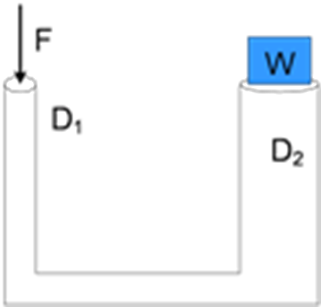a) 190 Newton b) 180 Newton c) 200 Newton d) 210 Newton
 Q.10 Given four bodies with each mass is 100 kg. The most little pressure at the bottom surface of the bodies is...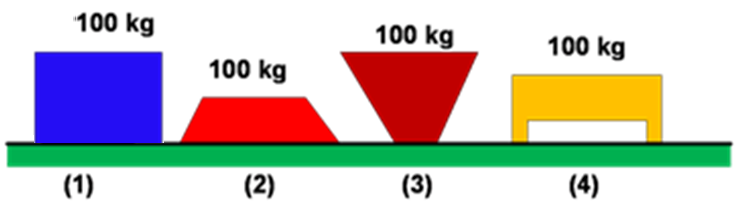a) 1 b) 2 c) 3 d) 4